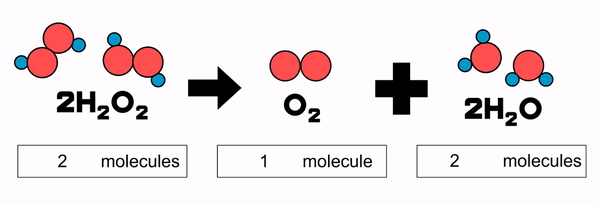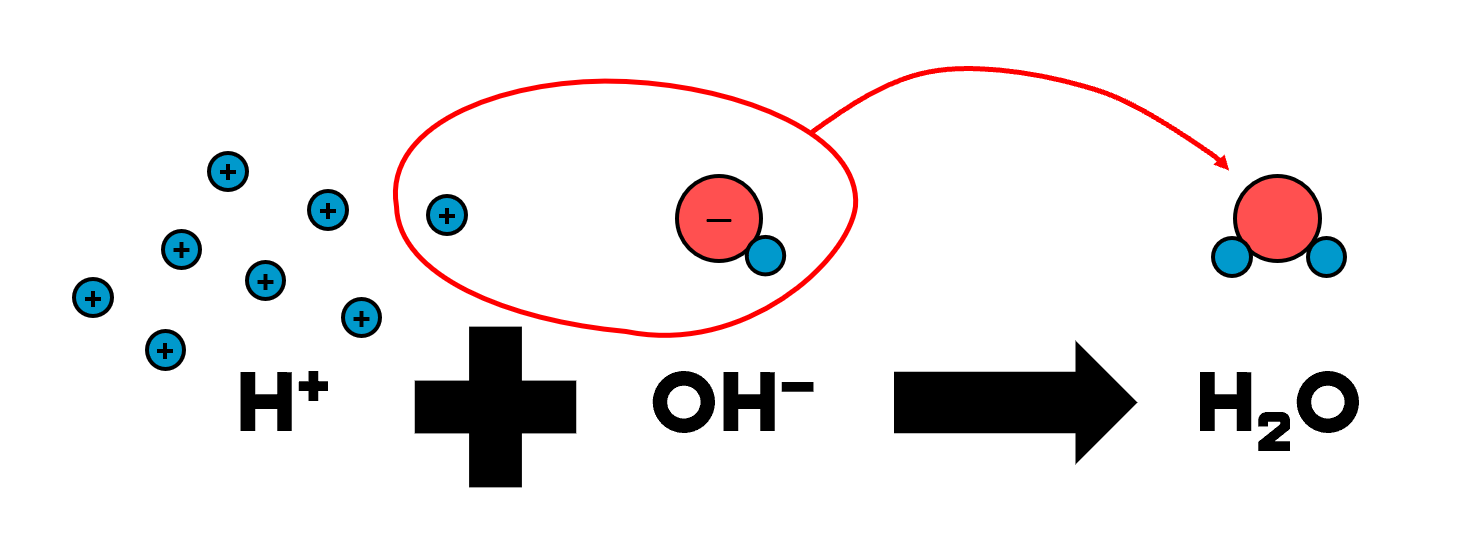## 1. Stoichio, the cheem word for ratio

Stoichiometry is a long, menacing word. But its meaning is simple: the ratio of the amount of reactants and products in a reaction. We get this ratio from the chemical equation.A balanced chemical equation showing the decomposition of liquid hydrogen peroxide, H2O2 into oxygen gas, O2 and liquid water, H2O

Let’s look at the chemical equation for the decomposition of hydrogen peroxide. There are two ways to think about the coefficients written before the chemical formulae.

Firstly, the coefficients give us the ratio in terms of number of molecules. For every 2 hydrogen peroxide molecule broken down, we get 1 molecule of oxygen and 2 molecules of water. Secondly, we can interpret the coefficients as the ratio in terms of moles. This means that for every 2 mol of hydrogen peroxide reacted, we get 1 mol of oxygen and 2 mol of water.

When we perform reactions in the laboratory, we deal with sizeable samples made up of large amounts of particles. Therefore, for chemical calculations, we will be using the second interpretation. We shall see the coefficients as mole ratio.

## 2. Using mole ratio to calculate the amount of product

We can use the number of moles of substances reacted to calculate the number of moles of products, and vice versa.

Let’s try to find out the amount of oxygen formed when we decompose 2 g of hydrogen peroxide.

• ### STEP 1: Write the chemical equation and highlight the substances you are interested in

2H2O2 (l) O2 (g) + 2H2O (l)

• ### STEP 2: Convert any given data to number of moles

Since we are given the mass of H2O2, divide it by its molar mass to find the number of moles.

Molar mass of H2O2 = 2 × 1 + 2 × 16 = 34 g/mol
No. of moles of H2O2 = 2 ÷ 34 = 0.0588 mol

• ### STEP 3: State the mole ratio

Ratio of O2 to H2O2 = 1:2

• ##### STEP 4: Use the mole ratio to find the number of moles of oxygen formed

No. of moles of O2 formed = 1/2 × 0.0588 = 0.0294 mol

## 3. No point adding excess, when the limiting reactants suppressNeutralisation reaction, whereby hydroxide ion is in short supply. It limits the number of water molecule formed.

Some reactions involve multiple reactants, like neutralisation. If we add one reactant in excess, the other reactant in short supply is the limiting reactant: it reacts fully and limits the amount of product formed.

In the neutralisation example above, hydrogen ions are in excess while hydroxide is limiting. No matter how many more hydrogen ions we add, there is only 1 hydroxide ion that can react with just 1 hydrogen ion. This limits the product to just 1 water molecule. We say that hydroxide ion is the limiting reactant here.

## 4. Identify limiting reactant to calculate the amount of products formed

We can identify the limiting reactant with a thought experiment. We randomly select a reactant that we suspect is limiting. Then we consider this: if it were fully reacted, would there be too much of the other reactant?

Let’s work through this with an example: find the amount of copper extracted when 2 g of copper(II) oxide was heated with 2 g of carbon.

• ### STEP 1: Write the chemical equation and highlight the substances you are interested in

2CuO (s) + C (s) ⟶ 2Cu (s) + CO2 (g)

• ### STEP 2: Convert any given data to number of moles

Molar mass of CuO = 64 + 16 = 80 g/mol
No. of moles of CuO =
2 ÷ 80 = 0.025 mol

Molar mass of C = 12 g/mol
No. of moles of C = 2 ÷
12 = 0.167 mol

• ### STEP 3: State the mole ratio of reactants

Ratio of C to CuO = 1:2

• ### STEP 4: Select a suspect and consider if it were reacted fully, would there be too much of the other reactant

Consider the case that CuO were completely reacted:

No. of moles of C used = 1/2 × 0.025 = 0.0125 mol

There was only enough CuO to react with 0.0125 mol of C, which was less than the 0.167 mol of C provided. Therefore, CuO was limiting and C was in excess.

• ### STEP 5: State the mole ratio of limiting reactant to product

Ratio of CuO to Cu = 2:2 = 1:1

• ### STEP 6: Use the limiting reactant to calculate the amount of product formed

No. of moles of Cu formed = 1/1 × 0.0250 = 0.0250 mol

## 5. Desperate to separate

Since the excess reactant is only partially reacted, the unreacted portion remains in the resulting mixture. Therefore, to purify the product, we will need to separate the unreacted portion.